# MP Board Class 10th Science Solutions Chapter 2 Acids, Bases and Salts

In this article, we will share MP Board Class 10th Science Solutions Chapter 2 Acids, Bases and Salts Pdf, These solutions are solved subject experts from the latest edition books.

## MP Board Class 10th Science Solutions Chapter 2 Acids, Bases and Salts

### MP Board Class 10th Science Chapter 2 Intext Questions

Intext Questions Page No. 18

Question 1.
You have been provided with three test tubes. One of them contains distilled water and the other two contain an acidic solution and a basic solution, respectively. If you are given only red litmus paper, how will you identify the contents of each test tube?

• Put the red litmus paper turn by turn in each of the three test tubes. The solution which turns the red litmus paper to blue will be a basic solution here, the blue litmus paper formed can now be used to test the acidic solution.
• Put this blue litmus paper in the remaining two test tubes one by one. The solution which turns the blue litmus paper to red will be the acidic solution.
• The solution which has no effect on any litmus paper will be neutral and hence it will be distilled water.

Intext Questions Page No. 22

Question 1.
Why should curd and sour substances not be kept in brass and copper vessels?
Curd and other sour substances are acidic in nature. So, when they are kept in brass and copper vessels, harmful products along with hydrogen gas are produced which spoil the food.Question 2.
Which gas is usually liberated when an acid reacts with a metal? Illustrate with an example. How will you test for the presence of this gas?
Hydrogen gas is usually liberated when an acid reacts with a metal. Let us illustrate it with the following examples:

1. Add some pieces of zinc granules into 5ml of a dilute solution of sulphuric acid (H2SO4).
2. Shake it well.
3. Pass the produced gas into a soap solution.
4. Now, soap bubbles are formed in the soap solution and these soap bubbles contain hydrogen.
5. Bring a burning candle near a gas-filled bubble. A candle burns with a pop sound. So, the following reaction takes place:
H2SO4(aq) + Zn(s) → ZnSO4(aq) + H26. We can test the evolved hydrogen gas by its burning with a pop sound when a candle is brought near the soap bubbles.

Question 3.
Metal compound A reacts with dilute hydrochloric acid to produce effervescence. The gas evolved extinguishes a burning candle. Write a balanced chemical equation for the reaction if one of the compounds formed is calcium chloride.Intext Questions Page No. 25

Question 1.
Why do HCl, HNO3 etc., show acidic characters in aqueous solutions while solutions of compounds like alcohol and glucose do not show acidic character?
HCl or HNO3 dissolve in water to form H+ or H3O+ ions in aqueous solutions which show their acidic character. The following reactions take place when HCl or HNO3 are mixed with water:
HCl(aq) → H+ + Cl
H+ + H2O → H3O+
On the other hand, when alcohol and glucose are mixed with water they do not dissolve to form ions due to the presence of hydrogen bonds and basic character. Hence, they do not show acidic character.

Question 2.
Why does an aqueous solution of acid conduct electricity?
In the aqueous solution, acid forms ions and these ions are conductor of electricity.

Question 3.
Why does dry HCl gas not change the colour of the dry litmus paper?
Dry HCl gas does not change the colour of the dry litmus paper because it has no hydrogen ions (H+) or hydronium (H3O+) ions in it.Question 4.
While diluting an acid, why is it recommended that the acid should be added to water and not water to the acid?
Mixing water to acid is an exothermic reaction. Hence while diluting an acid it is recommended that the acid should be added to water and not water to acid. If we mix water to acid explosion occurs and burning take place.

Question 5.
How is the concentration of hydronium ions (H3O+) affected when a solution of an acid is diluted?
Concentration of hydronium ions (H3O+) decreases and becomes weak. In this way concentration of hydronium ion affects when a solution of acid is diluted.

Question 6.
How is the concentration of hydroxide ions (OH) affected when excess base is dissolved in a solution of sodium hydroxide?
When excess base is dissolved in a solution of sodium hydroxide concentration of OH Hydroxide ion is more.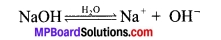Intext Questions Page No. 28

Question 1.
You have two solutions, A and B. The pH of solution A is 6 and pH of solution B is 8. Which solution has more hydrogen ion concentration? Which of this is acidic and which one is basic?
Solution ‘A’ is acidic because pH of the solution A is 6 which is less than 7 while solution ‘B’ is basic because pH of the solution ‘B’ is 8 which is greater than 7. Solution ‘A’ has more hydrogen ion concentration in comparison to solution ‘B’ because solution ‘A’ is acidic.

If the pH value is less than 7, it represents an acidic solution.
If the pH value is more than 7, it represents a base.
It the pH – 6 is acidic it has more concentration of ions than pH-8 which is a base.

Question 2.
What effect does the concentration of H+(aq) ions have on the nature of the solution?
If the concentration of H+(aq) ions is increased then the solution becomes acidic and if the concentration of H+(aq) ions is decreased then the solution becomes basic in nature.

Question 3.
Do basic solutions also have H+(aq) ions? If yes, then why are these basic?
Yes. H+ ions are always present in basic solution. Concentration of Basic is more than OH ions.

Question 4.
Under what soil condition do you think a farmer would treat the soil of his fields with quick lime (calcium oxide) or slaked lime (calcium hydroxide) or chalk (calcium carbonate)?
If the soil is acidic and improper for cultivation, then to increase the basicity of soil, the farmer would treat the soil with quick lime or slaked lime or chalk.

Intext Questions Page No. 33

Question 1.
What is the common name of the compound CaOCl2?
Bleaching powder.

Question 2.
Name the substance which on treatment with chlorine yields bleaching powder.
Dry slaked lime or calcium hydroxide.

Question 3.
Name the sodium compound which is used for softening hard water.
Sodium carbonate.
Na2CO3, 10 H2O is the compound of sodium to soften hard water.Question 4.
What will happen if a solution of sodium hydrocarbon is heated? Give the equation of the reaction involved.
When sodium hydrocarbon is heated then sodium carbonate and water are formed along with the evolution of carbon dioxide gas.
2NaHCO3 → Na2CO3 + H2O + CO2

Question 5.
Write an equation to show the reaction between Plaster of Paris and water.
Plaster of Paris reacts with water to form gypsum.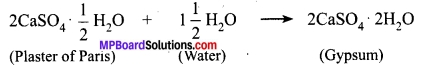### MP Board Class 10th Science Chapter 2 Ncert Textbook Exercises

Question 1.
A solution turns red litmus blue, its pH is likely to be
(a) 1
(b) 4
(c) 5
(d) 10
(d) 10
Bases turn red litmus to blue. pH value of 7 is greater than 7. Hence this solution changes red litmus to blue.

Question 2.
A solution reacts with crushed egg-shells to give a gas that turns lime-water milky. The solution contains
(a) NaCl
(b) HCl
(c) LiCl
(d) KCl
b) the solution contains HCl.

Question 3.
10 ml of a solution of NaOH is found to be completely neutralised by 8 ml of a given solution of HCl. If we take 20 ml of the same solution of NaOH, the amount of HCl solution (the same solution as before) required to neutralise it will be
(a) 4 ml
(b) 8 ml
(c) 12 ml
(d) 16 ml
d) 16 mL HCl solution is required.

Question 4.
Which one of the following types of medicines are used for treating indigestion?
(a) Antibiotic
(b) Analgesic
(c) Antacid
(d) Antiseptic
c) Antacid is used to treat indigestion.

Question 5.
Write word equations and then balanced equations for the reaction taking place when:
(a) dilute sulphuric acid reacts with zinc granules.
(b) dilute hydrochloric acid reacts with magnesium ribbon.
(c) dilute sulphuric acid reacts with aluminium powder.
(d) dilute hydrochloric acid reacts with iron filings.
a) Sulphuric acid + Zinc ➝ zinc Sulphate + Hydrogen
H2SO(aq) + Zn(s) ➝ ZnSO4(aq) + H2(g)
b) hydro Chloric acid + magnesium ➝ Magnisium Chloride + Hydrogen
2HCl(aq) + Mg(s) ➝ MgCl2(aq) + H2(g)
(c) Sulphuric Hydrigen Sulphate + Aluminium ➝ Aluminium + Hydrogen Chloride
3H2SO2(aq) + Mg(s) ➝ MgCl2(aq) + H2(g)
d) Hydrochloric acid  + Iron ➝ Ferric + Hydrogen
6HCl(aq) + 2Fe(s) ➝ 2FeCl2(aq) + 3H2(g)

Question 6.
Compounds such as alcohols and glucose also contain hydrogen but are not categorised as acids. Describe an activity to prove it.
Experiment: Fix two nails on the cork and keep this in 100 ml beaker. Two nails are fixed to 6 volt battery, bulb and switch. Then pour some dilute HCl in the beaker and switch on the current. Repeat the experiment separately with glucose and alcohol solutions.
Observation: Bulb glows in HCl solution but do not glows in glucose solution.
Result: HCl ➝ H+ and Cl- ions.
These ions conduct electricity and bulb glows.
By this experiment we conclude that All acids contain Hydrogen.

Question 7.
Why does distilled water not conduct electricity, whereas rainwater does?
Distilled water cannot conduct electricity because it does not contain ions while rainwater conducts electricity as it contains ions due to the presence of dissolved salts in it.

Question 8.
Why do acids not show acidic behavior in the absence of water?
Acids do not show acidic property in the absence of water. Because Hydrogen ions dissociates in presence of water. Hydrogen ions are responsible for acidic nature.

Question 9.
Five solutions A, B, C, D and E when tested with universal indicator showed pH as 4, 1, 11, 7 and 9, respectively. Which solution is
(a) Neutral?
(b) Strongly alkaline?
(c) Strongly acidic?
(d) Weakly acidic?
(e) Weakly alkaline?
Arrange the pH in increasing order of hydrogen-ion concentration.
a) Neutral ➝ solution D ➝ pH value of pH is 7.
b) strongly alkaline ➝ solution C ➝ pH is 11
c) strongly acidic ➝ solution B ➝ pH is 1
d) weakly acidic ➝ solution A ➝ pH is 4
e) weakly alkaline ➝ solution E —> pH is 9
We can arrange the pH in increasing order of hydrogen ion concentration as 11 < 9 <7 <4<1Question 10.
Equal lengths of magnesium ribbons are taken in test tubes A and B. Hydrochloric acid (HCl) is added to test tube A, while acetic acid (CH3COOH) is added to test tube B. Amount and concentration taken for both the acids are same. In which test tube will the fizzing
occur more vigorously and why?
The fizzing will occur strongly in test tube A, in which hydrochloric acid (HCl) is added. This is because HCl is a stronger acid than CH3COOH and therefore, produces hydrogen gas at a faster speed due to which fizzing occurs.

Question 11.
Fresh milk has a pH of 6. How do you think the pH will change as it turns into curd? Explain your answer.
pH value of fresh milk is 6, but when it converts into curd value of pH decreases because curd is acidic. Hence this value is becoming less.

Question 12.
A milkman adds a very small amount of baking soda to fresh milk.

1. Why does he shift the pH of the fresh milk from 6 to slightly alkaline?
2. Why does this milk take a long time to set as curd?

1. The milkman shifts the pH of the fresh milk from 6 to slightly alkaline because, in alkaline condition, milk does not set as curd easily. Hence, it does not get spoiled for longer period of time, in which he can sell it to make a profit.
2. Since this milk is slightly basic than usual milk, acids produced to set the curd are neutralised by the base. Therefore, it takes a longer time for the curd to set which is usually acidic.

Question 13.
Plaster of Paris should be stored in a moisture-proof container. Explain why?
The Plaster of Paris should be stored in a moisture-proof container as it absorbs water from moisture and turns into a hard substance (Gypsum) as shown in the following chemical equation:Question 14.
What is a neutralisation reaction? Give two examples.
The reaction between an acid a base to give salt and water is known as a neutralisation reaction.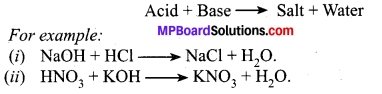Question 15.
Give two important uses of washing soda and baking soda.
1. Washing Soda:
(a) This is used in glass, soap and paper industries,
(b) It is used for removing permanent hardness of water.

2. Baking Soda:
(a) This is used cooking mixture of Baking soda and acid (tartaric acid – weak acid) is called Baking powder. When it is heated or combined with water. CO2 is evolved and soften the bread.
(b) It is also used in soda-acid fire extinguishers.

### MP Board Class 10th Science Chapter 2 Additional Important Questions

MP Board Class 10th Science Chapter 2 Multiple Choice Questions

Question 1.
The range of a pH scale is:
(a) 1 – 10
(b) 1 – 100
(c) 0 – 14
(d) 1 – 14
(c) 0 – 14

Question 2.
pH is defined as:
(a) The logarithm of hydrogen ion concentration
(b) The negative logarithm of hydrogen ion concentration
(c) Hydrogen ion concentration
(d) None of the above
(a) The logarithm of hydrogen ion concentrationQuestion 3.
Which of the following solution will have pH = 7?
(a) Tea
(b) The salt solution in distilled water
(c) Hydrochloric acid solution
(d) Water distilled with chlorine gas.
(b) The salt solution in distilled water

Question 4.
Which colour indicate neutral solution on a pH paper?
(a) Brown
(b) Green
(c) Purple
(d) White or transparent
(b) Green

Question 5.
A solution is acidic if:
(a) it releases H+ ions in the solution.
(b) it has a pH of less than 7.
(c) it has dark red, orange or greenish-yellow colour on a pH paper.
(d) all of the above.
(d) all of the above.

Question 6.
Which of the following solutions will have pH < 7?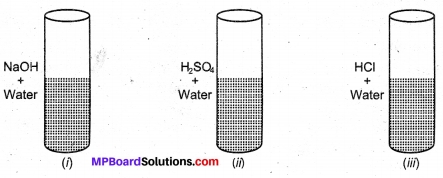Choose correct combination:
(a) (i) and (ii)
(b) (ii) and (iii)
(c) (i) and (iii)
(d) All of the above.
(b) (ii) and (iii)

Question 7.
“p” in pH stands for:
(a) Phosphorus
(b) Potenz
(c) Potential
(d) Polarity
(b) Potenz

Question 8.
Four different jars A, B, C, D contains hydrochloric acid, black coffee, ammonia and soap solution. Choose the order of decreasing acidic strength.(a) A > B > C > D
(b) B > C > A > D
(c) D > C > B > A
(d) D = C > B > A
(a) A > B > C > D

Question 9.
How can we find the pH of a solution?
(a) By dipping pH paper in it.
(b) By dropping some solution over pH paper.
(c) By heating pH paper in vapours of solution.
(d) By pouring all the solution over pH paper.
(a) By dipping pH paper in it.

Question 10.
In a class, while doing practical on different solutions, four students give their observations. Which student/s has/ve given a correct explanation if their observation is as follows?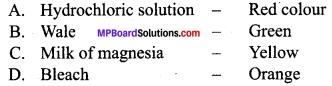Choose the correct combination of students with wrong observations:
(a) A and B
(b) C and D
(c) A, B, C
(d) All students
(b) C and D

Question 11.
Lemon juice gives orange colour over pH paper. What is its nature?
(a) Strong Acid
(b) Basic
(c) Neutral
(d) Moderate acid
(a) Strong Acid

Question 12.
Pure water has a pH = 7, while distilled water has pH = 8 – 10, it represents that distilled water is:
(a) Slightly basic
(b) Strong base
(c) Mild acid
(d) Strong acid
(a) Slightly basic

Question 13.
On a pH paper, pH values of 1 and 8 are represented by colours:
(a) Yellow and Orange
(b) Purple and Greenish
(c) Red and Bluish Green
(d) Green and Red
(c) Red and Bluish Green

Question 14.
Which of the following acids gives a dark red colour?
(a) Lemon juice
(b) Hydrochloric acid
(c) Acetic acid
(d) Nitric acid
(b) Hydrochloric acid

Question 15.
How pOH can by represented?
(a) -Log [H+] = pOH
(b) -Log [H] = pOH
(c) -Log [pH] = pOH
(d) -Log [OH] = pOH
(d) -Log [OH] = pOH

Question 16.
If [H+] is 1.0 × 10-9 mole, what will be pH of solution?
(a) 10-9
(b) 1
(c) 9
(d) -9
(c) 9

Question 17.
What is the nature of citric acid?
(a) Basic
(b) Acidic
(c) Neutral
(d) Both a and b
(b) Acidic

Question 18.
What is the nature of Sodium Hydroxide?
(a) Basic
(b) Acidic
(c) Neutral
(d) Unpredictable
(a) Basic

Question 19.
pH > 7 represents?
(a) Basic solutions
(b) Acidic solutions
(c) Neutral solution
(d) All
(a) Basic solutions

Question 20.
pH < 7 represents?
(a) Basic solutions
(b) Acidic solutions
(c) Neutral solution
(d) All
(b) Acidic solutions

Question 21.
Hydrogen ion concentration for pure water is:
(a) 7
(b) 10-7
(c) 10-7 mole/litre
(d) 107 moles/litre
(c) 10-7 mole/litre

MP Board Class 10th Science Chapter 2 Very Short Answer Type Questions

Question 1.
How can pH be represented using log?
pH = – log [H+].

Question 2.
What is the range of pH on pH paper?
0 – 14.

Question 3.
What is the pH of a strong acid?
0 – 2.

Question 4.
Why is water neutral?
On dissociation, water has equal numbers of H+ and OH ions. So, it does not go through any change and remains neutral.

Question 5.
What is the pH of concentric HCl?
1 – 2.

Question 6.
What will be the colour of pH paper when coffee is poured over it?
Reddish as it is slightly acidic in nature.

Question 7.
Which is more acidic-lemon juice or baking powder?
Lemon juice.

Question 8.
What is the best medium to check any chemical’s nature?
Water.

Question 9.
What is the universal solvent?
Aqua regia.

Question 10.
What kind of reactions are neutralisation reactions?
Any reaction between an acid and a base to form salt and water is called neutralisation reaction.

Question 11.
Which solution is considered to be neutral?
Solutions with no acidity or alkalinity are neutral. Acids and bases are present in equal amounts.Question 12.
Write the formula of brine and bleaching powder.
NaCl and CaOCl2.

Question 13.
Name two products which we can be obtained by chemical processing of common salt.
Baking soda and bleaching powder.

Question 14.
What is the common name of sodium hydrogen carbonate?
Baking soda.

Question 15.
Write the formula for washing soda.
Na2CO3.10H2O.

Question 16.
What is the source of naturally occurring acid lactic acid?
Curd.

Question 17.
Which acid is present in tomato?
Oxalic acid.

Question 18.
Name two olfactory indicators.
Vanilla and clove.

Question 19.
What is the colour of methyl orange in acidic solution?
Red.

Question 20.
What is the colour of phenolphthalein in basic medium?
Pink.

MP Board Class 10th Science Chapter 2 Short Answer Type Questions

Question 1.
How acids are different from bases when dissolved in water?
Acids on dissolving in water produce H+ ions while the base produces OHions.

Question 2.
Name two indicators and write their colour in different mediums.

 Name of indicator Colour in acidic medium Colour in basic medium Methyl Orange Red Yellow Pink Phenolphthalein Colourless Pink

Question 3.
Name any three hydrated salts.

1. Barium chloride, BaCl2. 2H2O.
2. Copper sulphate, CuSO4. 5H2O.
3. Ferrous sulphate, FeSO4. 6H2O.

Question 4.
Give an equation of neutralisation reaction.Question 5.
What causes acidity in our body? How can it be cured?
Our stomach produces hydrochloric acid which helps in digestion of food. During indigestion, the stomach produces too much acid and this causes pain and irritation. This can be cured by using bases called antacids.

Question 6.
What is the result of the reaction between an acid and a metal?
Corresponding salt is formed with the evolution of hydrogen gas when a metal reacts with acid.

Question 7.
Write two important uses of pH in everyday life. Also, give an example.
pH balance and its particular range of maintenance are very important in nature because it affects animal and plant life very much.

For example:

1. Curd formation: Atmospheric bacteria change the pH of milk which causes the curd formation.
2. Aids in digestion: Slight acidic conditions in the stomach due to the presence of hydrochloric acid aids in the digestion of food.

Question 8.
Give examples of two acids and bases present in nature.

1. Acids: Citric acid, acetic acid.
2. Bases: Calcium carbonate, sodium hydroxide.

Question 9.
Discuss the various types of salts.
There are three types of salts:

1. Neutral salts: Salts formed by the mixing of strong acid and strong base, e.g., NaCl, K2SO4 etc.
2. Acidic salts: Salts formed by the mixing of a strong acid and weak base e.g., NH4Cl, CaSO4.
3. Basic salts: Salts formed by the mixing of a strong base and weak acid e.g., Na2CO3, CH3COONa etc.

Question 10.
Common salt acts as raw material for many important daily use chemicals. Name some of them and also write their chemical formula.
Sodium hydroxide (NaOH), Baking Soda (NaHCO3), Washing soda (Na2CO3. 10H2O) etc.

Question 11.
What are the products of Chlor-alkali process?
The products of Chlor-alkali process are chlorine and sodium hydroxide.

Question 12.
Name two uses of each of the given salts:

1. Bleaching powder
2. Baking soda

Use of given salts are:
1. Bleaching powder:

• It is used as an oxidising agent in chemical industries.
• It is used for disinfecting water to make it free of germs.

2. Baking soda:

• It is used in soda acid fire extinguishers.
• It is an ingredient in antacids.

Question 13.
What is the water of crystallisation?
The water of crystallisation is the fixed number of water molecules present in one formula unit of salt.

Question 14.
Give reaction to show the formation of sodium zincate?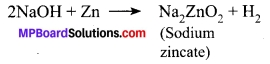Question 15.
Name the products of electrolysis of brine and also give on use of each.
Chlorine gas, H2 gas, and sodium hydroxide are the products of electrolysis of brine:

1. Use of chlorine gas: It is used as a disinfectant.
2. Use of H2 gas: It is used in the manufacture of ammonia.
3. Use of sodium hydroxide: It is used for the manufacture of soaps and detergents.

MP Board Class 10th Science Chapter 2 Long Answer Type Questions

Question 1.
Discuss the nature of the solution and its type in brief and also explain the strength of a solution.
Nature of the solution:
When a solute is dissolved solvent (generally water), it shows different kind of nature with regard to its reactivity and solubility. On the basis of removal of H+ ion or OH the solution is formed. The types of solutions are divided as follows:Strength of solution is determined by the,

1. Speed of reactivity i.e., how fast the ions are found dissociated.
2. Amount of ions (H+ or OH ) released or their ion concentration.

Question 2.
Give one example in each case:
(a) a weak mineral acid.
(b) a base which is not an alkali.
(c) a hydrogen-containing compound which is not an acid.
(d) a basic oxide soluble in water.
(e) a basic oxide insoluble in water.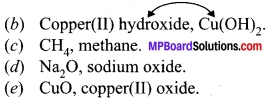### MP Board Class 10th Science Chapter 2 Textbook Activities

Class 10 Science Activity 2.1 Page No. 18

1. Collect the following solutions from the science laboratory – hydrochloric acid (HCl), sulphuric acid (H2SO4), nitric acid (HNO3), acetic acid (CH3COOH), sodium hydroxide (NaOH), calcium hydroxide (Ca(OH)2], potassium hydroxide (KOH), magnesium hydroxide [Mg(OH)2] and ammonium hydroxide (NH4OH).
2. Put a drop of each of the above solutions on a watch-glass one by one and test with a drop of the indicators shown in the table.
3. What change in colour did you observe with red litmus, blue litmus, phenolphthalein and methyl orange solutions for each of the solutions taken?
4. Tabulate your observations in the table.

Result: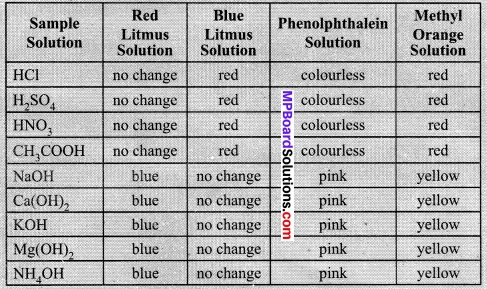Class 10 Science Activity 2.2 Page No. 18,19

1. Take some finely chopped onions in a plastic bag along with some strips of clean cloth. Tie up the bag tightly and leave overnight in the fridge. The cloth strips can now be used to test for acids and bases.
2. Take two of these cloth strips and check their odour.
3. Keep them on a clean surface and put a few drops of dilute HCl solution on one strip and a few drops of dilute NaOH solution on the other.
4. Rinse both cloth strips with water and again check their odour.
6. Now take some dilute vanilla essence and clove oil and check their odour.
7. Take some dilute HCl solution in one test tube and dilute NaOH solution in another. Add a few drops of dilute vanilla essence to both test tubes and shake well. Check the odour once again and record changes in odour, if any.
8. Similarly, test the change in the odour of clove oil with dilute HCl and dilute NaOH solution and record your observations.

Observations:

1. On putting the cloth strip into dilute.HCl solution, the red colour of cloth strip changes to pale red.
2. On putting the cloth strip into NaOH solution, the red colour of strip changes to green in colour.

Odour test:

1. Add vanilla essence in dilute NaOH.
2. Add vanilla essence in dilute HCl.
3. Add clove oil in to dilute HCl.
4. Add clove oil in dilute NaOH.

Result:

1. No smell found.
2. The smell of vanilla exists.
3. The smell of clove present.
4. The smell of clove exists.

Class 10 Science Activity 2.3 Page No. 19,20

Caution:

1. This activity needs the teacher’s assistance.
2. Set the apparatus as shown in Figure.
3. Take about 5 ml of dilute sulphuric acid in a test tube and add a few pieces of zinc granules to it.
4. What do you observe on the surface of zinc granules?
5. Pass the gas being evolved through the soap solution.
6. Why are bubbles formed in the soap solution?
7. Take a burning candle near a gas-filled bubble.
8. What do you observe?
9. Repeat this Activity with some more acids like HCl, HNO3 and CH3COOH.
10. Are the observations in all the cases the same or different?Observations:

1. 5 ml of dilute sulphuric acid + zinc granules → On the surface of zinc granules + bubbles of hydrogen gas are formed.
2. On passing the gas evolved through a soap solution → bubbles are formed due to low surface tension of soap solution. On taking a burning candle near a gas-filled bubbles → gas burns with a pop sound.
3. All other acids HCl, HNO3 and CH3COOH show the same observation.

Class 10 Science Activity 2.4 Page No. 20

1. Place a few pieces of granulated zinc metal in a test tube.
2. Add 2 ml of sodium hydroxide solution and warm the contents of the test tube.
3. This activity needs the teacher’s assistance.
4. Set the apparatus as shown in Figure.
5. Take about 5 ml of dilute sulphuric acid in a test tube and add a few pieces of zinc granules to it.
6. What do you observe on the surface of zinc granules?
7. Pass the gas being evolved through the soap solution.
8. Why are bubbles formed in the soap solution?
9. Take a burning candle near a gas-filled bubble.
10. What do you observe?
11. Repeat this Activity with some more acids like HCl, HNO3 and CH3COOH.
12. Are the observations in all the cases the same or different?

Observations:
Reaction:
2NaOH + Zn → Na2ZnO3 + H2

Conclusion:
Bubbles of hydrogen gas are formed.

Class 10 Science Activity 2.5 Page No. 20

1. Take two test tubes, label them as A and B.
2. Take about 0.5 g of sodium carbonate (Na2CO3) in test tube A and about 0.5 g of sodium hydrogen carbonate (NaHCO3) in test tube B.
3. Add about 2 ml of dilute HCl to both the test tubes.
4. What do you observe?
5. Pass the gas produced in each case through lime water (calcium hydroxide solution) as shown below and record your observations.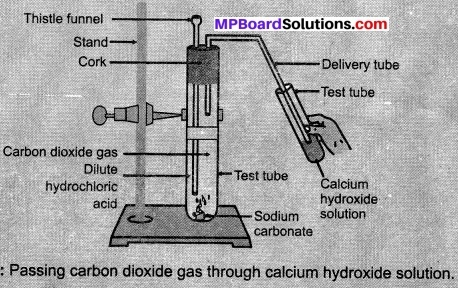Observations:
Test Tube A:
Reaction:
Na2CO3(s) + 2HCl(aq) → 2NaCl(aq) + H2O(l) +CO2(g)

Test Tube B:
Reaction:
NaHCO3(s) + HCl(aq) → NaCl(aq) + H2O(l) + CO2(g)
On passing CO2 gas through lime water it turns milky because insoluble white precipitate of CaCO3 is formed as shown below: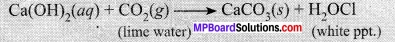On passing excess gas through lime water, it becomes colourless.Class 10 Science Activity 2.6 Page No. 21

1. Take about 2 ml of dilute NaOH solution in a test tube and add two drops of phenolphthalein solution.
2. What is the colour of the solution?
3. Add dilute HCl solution to the above solution drop by drop.
4. Is there any colour change for the reaction mixture?
5. Why did the colour of phenolphthalein change after the addition of an acid?
6. Now add a few drops of NaOH to the above mixture.
7. Does the pink colour of phenolphthalein reappear?
8. Why do you think this has happened?

Observations:

1. Add 2 drops of phenolphthalein solution into NaOH solution → colour of the solution is pink.
2. Now add dilute HCl solution drop by drop.
3. Now, reaction mixture changes to colourless.
4. This is because neutralisation of HCl and NaOH takes place.
5. Now, add NaOH (few drops) → Pink colour reappears.

Class 10 Science Activity 2.7 Page No. 21

1. Take a small amount of copper oxide in a beaker and add dilute hydrochloric acid slowly while stirring.
2. Note the colour of the solution. What has happened to the copper oxide?

Observations:

1. Add dilute HCl to copper oxide solution:
2. Colour of the solution turns into green and CuCl2 dissolves.
3. The blue-green colour formed due to formation of copper (II) chloride,
CuO + 2HCl → CuCl + H2O

Class 10 Science Activity 2.8 Page No. 22

1. Take solutions of glucose, alcohol, hydrochloric acid, sulphuric acid etc.
2. Fix two nails on a cork and place the cork in a 100 ml beaker.
3. Connect the nails to the two terminals of a 6-volt battery through a bulb and a switch, as shown in Figure.1. Now pour some dilute HCl in the beaker and switch on the current. Repeat with dilute sulphuric acid.
2. What do you observe?
3. Repeat the experiment separately with glucose and alcohol solutions.
4. What do you observe now?
5. Does the bulb glow in all cases?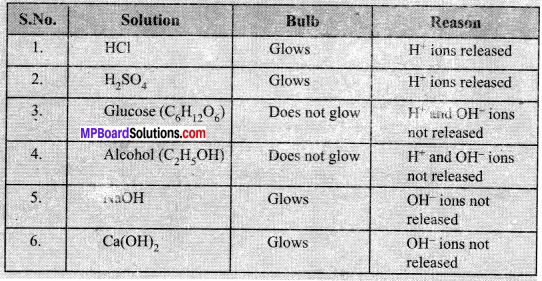Class 10 Science Activity 2.9 Page No. 23

1. Take about 1g solid NaCl in a clean and dry test tube and set up the apparatus as shown in Figure.
2. Add some concentrated sulphuric acid to the test tube.
3. What do you observe? Is there a gas coming out of the delivery tube?
4. Test the gas evolved successively with dry and wet blue litmus paper.
5. In which case does the litmus paper change colour?
6. On the basis of the above Activity, what do you infer about the acidic character of:
• Dry HCl gas?
• HCl solution?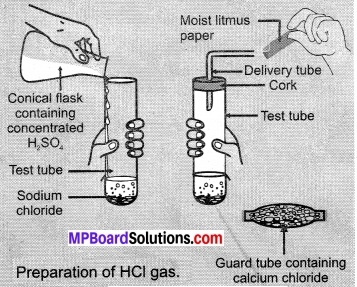Note to teachers:
If the climate is very humid, you will have to pass the gas produced through a guard tube (drying tube) containing calcium chloride to dry the gas.

Observations:

1. Adding concentrated H2SO4 to test tube containing NaCl leads to the production of HCl gas. Now, testing this gas with litmus paper following were recorded,
2. HCl gas passes → Colour change
3. Dry litmus paper → No change
4. Wet litmus paper → Blue litmus turns red

Thus, only HCl solution release H+ ions and acidic property exist due to H+ ions.

Class 10 Science Activity 2.10 Page No. 24

1. Take 10 ml water in a beaker.
2. Add a few drops of concentrated H2SO4 to it and swirl the beaker slowly.
3. Touch the base of the beaker.
4. Is there a change in temperature?
5. Is this an exothermic or endothermic process?
6. Repeat the above Activity with sodium hydroxide pellets and record your observations.

Observations:

1. Add a few drops of concentrated H2SO4 to the water in a beaker → it becomes hot as the reaction is highly exothermic.
2. Now, add NaOH pellets to water → the beaker becomes hot, the reaction is exothermic.

Class 10 Science Activity 2.11 Page No. 26

1. Test the pH values of solutions given in the table.
3. What is the nature of each substance on the basis of your observations?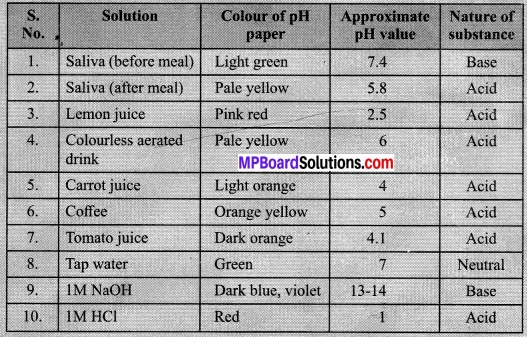Class 10 Science Activity 2.12 Page No. 27

1. Put about 2 g soil in a test tube and add 5 mL water to it.
2. Shake the contents of the test tube.
3. Filter the contents and collect the filtrate in a test tube.
4. Check the pH of this filtrate with the help of universal indicator paper.
5. What can you conclude about the ideal soil pH for the growth of plants in your region?

Observations: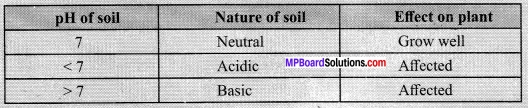Class 10 Science Activity 2.13 Page No. 28,29

1. Write the chemical formulae of the salts given below.
Potassium sulphate, sodium sulphate, calcium sulphate. magnesium sulphate, copper sulphate, sodium chloride, sodium nitrate, sodium carbonate and ammonium chloride.
2. Identify the acids and bases from which the above salts may be obtained.
3. Salts having the same positive or negative radicals are said to belong to a family. For example. NaCl and Na2SO4 belong to the family of sodium salts. Similarly, NaCl and KCl belong to the family of chloride salts. How many families can you identify among the salts given in this Activity?Class 10 Science Activity 2.14 Page No. 28

1. Collect the following salt samples – sodium chloride, potassium nitrate, aluminium chloride, zinc sulphate, copper sulphate, sodium acetate, sodium carbonate and sodium hydrogen carbonate (some other salts available can also be taken).
2. Check their solubility in water (use distilled water only).
3. Check the action of these solutions on litmus and find the pH using a pH paper.
4. Which of the salts are acidic, basic or neutral?
5. Identify the acid or base used to form the salt.
6. Report your observations in the table.Class 10 Science Activity 2.15 Page No. 32

1. Heat a few crystals of copper sulphate in a dry boiling tube.
2. What is the colour of the copper sulphate after heating?
3. Do you notice water droplets in the boiling tube? Where have these come from?
4. Add 2-3 drops of water on the sample of copper sulphate obtained after heating
5. What do you observe? Is the blue colour of copper sulphate restored?

Observations:

1. On heating blue crystals of copper sulphate, it becomes colourless or white and few drops of water are seen on test tube due to condensation of water of crystallisation.
2. On adding few drops of water to heated anhydrous copper sulphate, the blue colour of copper sulphate reappear.